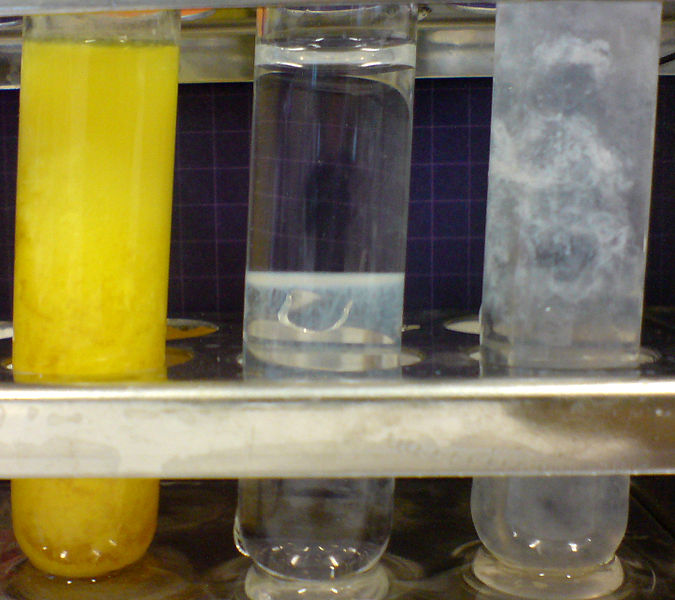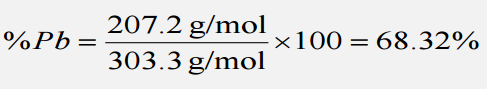Are you more of a visual learner? Check out our online video lectures and start your chemistry course now for free!## Introduction to Ionic Reactions

Concentrations of solutions may be expressed in different forms. These include molarity, molality, % weight/volume, % weight/weight, % volume/volume, normality, titer, and much more. The most common concentration quantity for the ionic solution is the molarity. Molarity is expressed as the number of moles of solute per L of the solution. For example, to compute for the concentration of a sodium chloride solution that is prepared by dissolving 1 g of NaCl in 1.00 L of the solution, the following equation will be used.

Each atom that participates in an oxidation-reduction reaction is assigned an oxidation number that reflects its ability to acquire, donate or share electrons.

## Redox Reaction

Redox is a short-hand notation for reduction-oxidation. These reactions are named Redox reactions because the reaction involves two simultaneous steps which are reduction and oxidation reaction. These are reactions wherein electron transfer is involved between two chemical species. In the process, one of the reactants loses electrons, while the other species gain the electron. The charge is also conserved in the process.

## Oxidation State

Oxidation state, or number, is the total number of electrons that an atom has gained or has lost to form a chemical bond. Each atom participating in an oxidation-reduction reaction is assigned an oxidation state to determine the ability of each atom to accept, donate or share electrons. Changes in the oxidation states of the atom will reflect whether those atoms were reduced or oxidized in the process. Some general rules for assigning oxidation states are listed below.

1. The oxidation state of the atoms in a free, elemental form is zero.
2. The oxidation state of an element in monoatomic ions is equal to the charge of the ion.
3. For a neutral compound, the sum of the oxidation states of all atoms should be equal to zero. For a polyatomic ion, the sum of all oxidation states of the atoms in the structure of the ion is equal to the charge of the ion.
4. Fluorine has an oxidation state of -1 in all of its compounds.
5. Hydrogen has an oxidation state of +1 in all its compounds, except when combined with metals where its oxidation state is -1.
6. Oxygen can have a number of oxidation states depending on what other elements it is combined with. Generally, it has an oxidation state of -2, except for the following: A quick look at the position of an element in the periodic table can help to assign the oxidation state.
1. Peroxide: O has an oxidation state of -1 if it is in its peroxide state, O22-.
2. Superoxides: O has an oxidation state of -1/2 in its superoxide state, O2.
3. Oxygen has an oxidation state of +2 when combined with the more electronegative element F.
7. A quick look at the position of an element in the periodic table can help to assign the oxidation state.
1. Group 1A have an oxidation state of +1 in all of their compounds.
2. Group 2A have an oxidation state of +2 in all of their compounds.
3. Group 3A have an oxidation state of +3 in all of their compounds.
4. Group 5A elements have an oxidation state of -3 in binary compounds with metals, H, or with NH4+. For compounds of Group 5A elements with an element, it’s right in the periodic table where rules 3 and 4 may apply.
5. Group 6A elements below oxygen have an oxidation state of -2 in binary compounds with metals, H and NH4+.
6. Group 7A elements have oxidation states of -1 in binary compounds with metals, H and NH4+.

## Reducing and Oxidizing Agents

In a redox reaction, the compound that loses electrons are termed oxidized and is called the reducing agent. On the other hand, the compound that accepts electrons during the process is termed reduced and is called the oxidizing agent.

For example, in the reaction of Fe2+ ions with Ce4+ ions:
Fe2+  +   Ce4+  → Fe3+   +    Ce3+

We can write the reaction above into two half-reaction or steps:
Fe2+ →  Fe3+ + 1e               Oxidation
Ce4+  + 1 e →  Ce3+              Reduction

Fe2+ ions lose electrons to produce Fe3+. There is an increase in the oxidation state, and so the process is termed as oxidation. The electrons released by Fe2+ will then be accepted by the Ce4+ producing Ce3+. In the next half-reaction, the oxidation state of Ce becomes lower in the process of reduction. Fe2+ is oxidized in the process while Ce4+ is reduced. In this reaction, Fe2+ is the reducing agent, while Ce4+ is the oxidizing agent.

## Balancing Redox Reactions

Like any reactions, redox reactions follow the law of conservation of matter but aside from balancing the elements, overall charges in the reaction are also to be balanced. Balancing redox reactions may be achieved using the steps described below. As an example, consider the reaction of the permanganate ion, MnO4, and sulfurous acid, H2SO3.

1. The reaction will be divided into 2 half-reactions. To identify the half-reactions, you just need to look at the atoms which changed oxidation states in the reaction.

2. The next step is to balance the atoms beside H and O. Most of the time, these atoms are the ones oxidized or reduced. Since, in the example, there are no differences in the O and H atoms, the half-reactions are retained.

3. The O atoms are then balanced by adding water in either the reactant or the product side.

Al(OH)3 + 3HCI → 3H3O+ + AlCl3 (Base)

Al(OH)3 + OH → Al(OH)4- (Acid)

4. After balancing the O atoms, in most cases, there will be an excess of H-atom on one side. The H atoms are then balanced by adding H+ to either side of the reaction.

5. By this time, it will be noticed that the charges of the half-reactions are not balanced. The next step then is to balance the charges by adding electrons to either side of the reactions.

6. The next step is to add the two half-reactions to get the over-all balanced redox reaction. Since an electron product cannot be yielded in a reaction, each half-reaction will be multiplied by a certain factor so that the electrons will be balanced.

HNO(3aq) + H2O(l) → H3O+(aq) + NO3-(aq)

7. Chemical entities present in the reactant and the product sides of the reaction are canceled. No electron should be present in the final balanced reaction. If the reaction is in acidic medium, you may stop at step no. 7.

8. In a basic medium, the H+ ions are completely neutralized by adding OH- on both sides of the reaction. The OH- combined with the H+ will produce water.

## Other Ionic Reaction (Precipitation Reaction)

Some ionic reactions lead to the formation of a precipitate or a solid. The stability of the product enables the formation of insoluble precipitates. Precipitates are commonly formed from the interaction of a cation and an anion in solution. When the two ions react with each other, a precipitate may form or not. Below is a list of the common solubility rules.

## Solubility Rules

1. Common inorganic acids and some low-molecular-weight organic acids are completely soluble in water.
2. All common compounds of the Alkali metal cations (Li+, Na+, K+, Rb+, Cs+) and the ammonium ion, NH4+, are soluble in water.
3. Salts containing nitrates, NO3; acetates, CH3COO; chlorates, ClO3; and perchlorates, ClO4, are soluble in water.
4. Chlorides, Cl, are usually soluble in water except for AgCl, Hg2Cl2, and PbCl2. Bromides, Br, and Iodide, I, follow the same solubility rule as the chlorides, except the degree of solubility decreases with the increasing size of the anion. Fluorides, F, are generally soluble, except MgF2, CaF2, SrF2, BaF2 and PbF2.
5. Sulfates, SO42-, are soluble except PbSO4, BaSO4, and HgSO4. CaSO4, SrSO4, Ag2SO4, on the other hand, are moderately soluble.
6. Hydroxides, OH, are insoluble in water, except the alkali metal and heavy alkali earth metal hydroxides.
7. Carbonates, CO32-, phosphates, PO43-, and arsenates, AsO43-, are insoluble in water, except with alkali metals and NH4+ cations. MgCO3 is moderately soluble.
8. Sulfides, S2-, are insoluble in water, except with alkali metal, alkali earth metal, and ammonium cations.

Sometimes, there is a need to write the Net Ionic Equation (NIE) of a reaction. NIE involves showing the predominant form of a substance when it is in contact with water. For example, a completely soluble salt like NaCl will not exist as solid NaCl in a reaction with water as a solvent. Instead, it will be represented as its ions Na+, and Cl–. Using the NIE, we can emphasize how the precipitates are formed in a reaction.

For example, let us consider the reaction of an aqueous solution of KI and AgNO3. Based on the solubility rules, both compounds are soluble in water. This means both compounds dissociate in water to produce their ionic components. As soon as a drop of KI is added to the AgNO3 solution, a yellow precipitate was formed.

Following the solubility rules, it will only be the AgCl product that will stay as a solid as it is insoluble in water. Using the total ionic equation for the reaction, we can emphasize that it is only the AgCl that is insoluble in our reaction system.

Total ionic equation:

[K+(aq) + I(aq)] + [Ag+(aq) + NO3(aq)] – [K+(aq)+NO3(aq)] + Agl(s)

As you can see, there are ions that are present in both the reactant and product sides. These ions are called spectator ions and are canceled to obtain the net ionic equation.

Net ionic equation:

Ag+(aq) + I(aq) → Agl(s)

## Example Calculation

Imagine a 1.00 L sample of polluted water was analyzed for lead(II) ion, Pb2, by adding an excess of sodium sulfate to it. The mass of lead(II) sulfate that precipitated was 229.8 mg.

What is the mass of lead in a liter of water? Express the answer as mg of lead per liter of solution.

Na2SO4(aq) + Pb2+(aq) → 2Na+(aq) + PbSO4(s)

First, we must obtain the mass percentage of lead in lead(II) sulfate, by dividing the molar mass of lead by the loar mass of PbSO4, then multiplying by 100.

The, calculate the amount of lead in the PbSOprecipitated.Amount Pb in sample = 229.8 mg PbSO  x 0.6832 = 157.0 mg Pb

Learn. Apply. Retain.
Your path to achieve medical excellence.
Study for medical school and boards with Lecturio.(Votes: 4, average: 4.50)Loading...